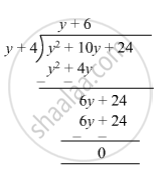SSC (English Medium) Class 8Maharashtra State Board
Share

# Divide and Write the Quotient and the Remainder. (Y2 + 10y + 24) ÷ (Y + 4) - SSC (English Medium) Class 8 - Mathematics

ConceptTo Divide a Polynomial by a Binomial

#### Question

Divide and write the quotient and the remainder.

(y2 + 10y + 24) ÷ (y + 4)

#### Solution

(y2 + 10y + 24) ÷ (y + 4)So, quotient = y + 6 and remainder = 0

Is there an error in this question or solution?

#### APPEARS IN

Balbharati Solution for Balbharati Class 8 Mathematics (2019 to Current)
Chapter 10: Division of Polynomials
Practice Set 10.2 | Q: 1 | Page no. 66
Solution Divide and Write the Quotient and the Remainder. (Y2 + 10y + 24) ÷ (Y + 4) Concept: To Divide a Polynomial by a Binomial.
S